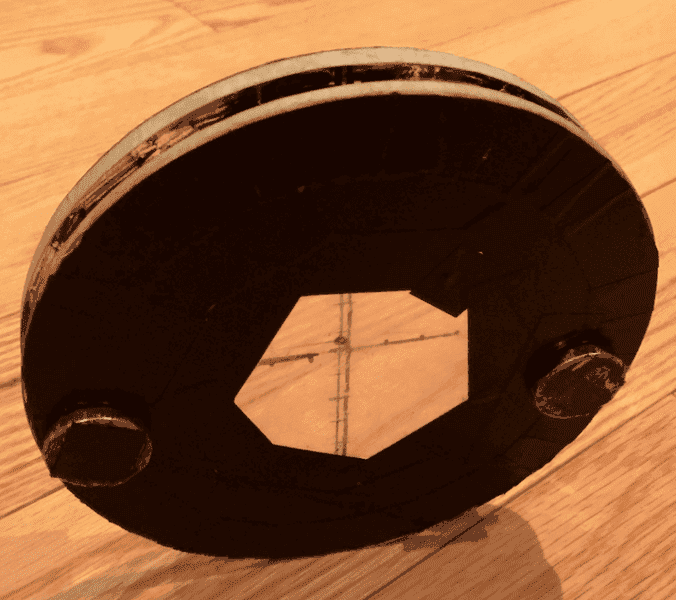# Calculating moment of inertia of a complicated system

iancheung228

## Homework Statement

I am currently doing a lab investigating the relationship between the moment of inertia and speed.
I have attached some pictures of my 'wheel' that is going to be rolled down a ramp. FYI i will be using a photogate to measure the time it takes the black strip to pass through. Initially, I thought the magnets were creating the 'hollow' part of the disc, but apparently i am wrong, and I am wondering how to calculate the moment of inertia of the magnet and then apply the moment of inertia to the equation mgh=1/2mv^2+I system (omega)^2.

## Homework Equations

MgH=v^2(m/2+Isystem/2r^2)
where M is the mass of the whole wheel system 0.369kg.
g is 9.8
H is the initial height on the ramp which is 0.105m
V is measured with a photogate ,0.10m/0.11746s= 0.85135m/s
radius of the circle is 8cm.
I of the system is (mr^2/2)+(mr^2/2)+8(mass of magnets (0.019kg(0.53m^2+2(0.078m^2))
where the mass of bgi wheel is 0.142kg and small wheel 0.074kg.

MgH=v^2(m/2+Isystem/2r^2)
where M is the mass of the whole wheel system 0.369kg.
g is 9.8
H is the initial height on the ramp which is 0.105m
V is measured with a photogate ,0.10m/0.11746s= 0.85135m/s
radius of the circle is 8cm.
I of the system is (mr^2/2)+(mr^2/2)+8(mass of magnets (0.019kg(0.53m^2+2(0.078m^2))
where the mass of bgi wheel is 0.142kg and small wheel 0.074kg.## The Attempt at a Solution

Homework Helper
Gold Member
I have attached some pictures of my 'wheel' that is going to be rolled down a ramp. FYI i will be using a photogate to measure the time it takes the black strip to pass through. Initially, I thought the magnets were creating the 'hollow' part of the disc, but apparently i am wrong, and I am wondering how to calculate the moment of inertia

Can you explain what you mean by "I thought the magnets were creating the 'hollow' part of the disc" ?

The moment of inertia of a composite is the sum of the moments of inertia of the parts. So break it down into parts and look up the moment of inertia of each. You will need to apply the parallel axis theorem.

iancheung228
Can you explain what you mean by "I thought the magnets were creating the 'hollow' part of the disc" ?

The moment of inertia of a composite is the sum of the moments of inertia of the parts. So break it down into parts and look up the moment of inertia of each. You will need to apply the parallel axis theorem.
yup i figured after having a conservation with another person on physics forum. is it true that theres increasing error as my magnet move towards the center of the wheel?